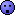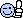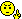# Math Is Fun Forum

Discussion about math, puzzles, games and fun.   Useful symbols: ÷ × ½ √ ∞ ≠ ≤ ≥ ≈ ⇒ ± ∈ Δ θ ∴ ∑ ∫  π  -¹ ² ³ °

You are not logged in.

## #1 Re: Help Me ! » Find A Linear Equation » 2023-11-26 14:13:36

Ok. You know your math. Terrific!

## #2 Re: Help Me ! » Sum of All Even Integers » 2023-11-24 13:15:45

I have moved on beyond the current topic. I will not post here unless it is totally needed.

## #3 Re: Help Me ! » Mathematical geography - homework » 2023-11-24 13:13:46

1. In what way are you going to receive help here if others solve your obvious homework problems?

2. We don't solve millions of questions per student.

3. Where are you stuck with question 1?

4. What effort have you made to solve question 1?

5. Post one question at a time.

## #4 Re: Help Me ! » Radicand Comes Out » 2023-11-24 13:12:45

Copy. Understood.

## #5 Re: Help Me ! » Product Promotion Application » 2023-11-24 13:11:39

Two points leads to finding the slope. We then use the point-slope formula
amnkb found.

Moving on....

Thanks.

## #7 Re: Help Me ! » Sum of All Even Integers » 2023-11-24 07:22:20

Bob wrote:

sologuitar

Your behaviour is becomong less and less acceptable. Let me try to explain so there's no misunderstanding..

(1) Each day, when I log in I see a long string of posts from you.  I find it very hard to remember which posts have been answered and which ones are still 'live'.  If I've given you help but it hasn't been enough to get you to your own answer I'll try to give more help, but, at the moment I'm getting lost in all the posts.

(2) Every time you respond to a post you quote the previous post.  That isn't helping either. It just increases the amount I have to wade through.  It is possible to respond without quoting the previous post. What would help is a dialogue consisting of the following:

(i) post asking for help on a question.

(ii) (a) response from you saying that's it, I've got it now or (b) please offer more help about ......

(iii) if (a) then the thread is finished if (b) then wait for additional help and go back to step (ii)

(3) amnkb has been trying to help you as well as me.  You were getting the whole solution from amnkb but you asked for this not to happen. I asked amnkb that whole solutions be hidden so you could still try to work it out for yourself. That is what is now happening! Each box is a hint and the 'contents' of the box is the answer you should have obtained for yourself.  That takes a lot of time to set up but gives you maximum help. It's exactly what you asked for.

So it is very ungenerous of you to say

I am tired of clicking on rectangles to see the answer.

If you want help you have to accept it in whatever form it is offered. If you don't like it then say nothing! You are coming close to being banned for upsetting other members and I have to keep the peace.

So how should you go forward from here?

Look at the hints offered by me and by  amnkb.  If it is enough for you, then say "thank you", and move on.  If it's not then say precisely what your problem is so someone can try to help. Stop being rude to the members who are giving up their time freely to help you.

Bob

1. I had no idea that posting a few questions is a problem.

2. No need to threaten banning me from the website.

4. I will try to adhere to your rules while searching for another math forum.

## #8 Re: Help Me ! » Sum of All Even Integers » 2023-11-22 17:00:33

amnkb wrote:
sologuitar wrote:

For any positive integer n, the sum of the first n positive integers equals n(n+1)/2. What is the sum of all the even integers between 99 and 301?

Can someone set this up for me?

Bob wrote:

The formula works for sets of integers that do not have any gaps so use the fact that evens are involved to get a continuous sequence of integers. ie:

100 + 102 + ... + 300 = 2 x (50 + 51 + ... 150)

So find ∑49 and ∑150, subtract and then [multiply by] 2.

sologuitar wrote:

I'm still a bit confused. Can you start this one for me?

working from bob's start & using his steps:

I will need to ignore your reply moving forward. I am tired of clicking on rectangles to see the answer.

## #9 Re: Help Me ! » Venn Diagram Mathematics » 2023-11-22 16:58:10

amnkb wrote:
sologuitar wrote:

In a class of 50 students, 20 play Hockey, 15 play Cricket and 11 play Football. 7 play both Hockey and Cricket, 4 play Cricket and Football and 5 play Hockey and football. If 18 students do not play any of these given sports, how many students play exactly two of these sports?

Can someone set this up for me?

Bob wrote:

Ok. Here's a start:

https://i.imgur.com/SrBFYZG.gif

sologuitar wrote:

I still can't come up with the equation. What is the correct equation to solve for x?

'set this up for me'

'what is correct eqn?'

Reply using LaTex form. I am tired of clicking on rectangles to see the answer.

## #10 Re: Help Me ! » Find A Linear Equation » 2023-11-22 16:56:31

amnkb wrote:
sologuitar wrote:

The relationship between Celsius (°C) and Fahrenheit (°F) degrees of measuring temperature is linear. Find a linear equation relating °C and °F if 0°C corresponds to 32°F and 100°C corresponds to 212°F.

amnkb wrote:

sologuitar wrote:

Which one? There are two formulas here. The slope formula or the point-slope formula or both?

Why can't you simply answer the question?
Why do I need to click to see the answer?

## #11 Re: Help Me ! » Access Ramp » 2023-11-22 16:52:39

amnkb wrote:
sologuitar wrote:

A wooden ramp is being built to reach a platform that sits 30 inches above the floor. The ramp drops 2 inches for every 25-inch run. Write a linear equation that relates the height y of the ramp above the floor to the horizontal distance x from the platform.

Can I please have a step by step explanation  of how this linear equation is form?

amnkb wrote:

sologuitar wrote:

What information in the application states that negative 2 over 25 is the slope or coefficient of x?

sry

Ok.

## #12 Re: Help Me ! » Mathematical geography - homework » 2023-11-22 10:54:49

amnkb wrote:
Bob wrote:

Wow!Is this a joke?  What school is expecting this as a hw assignment? And what age of student?

sologuitar wrote:

In what way are you going to receive help here if others solve your obvious homework problems?

glad these are obvious to you(my response is same as bob's)
youve said when you dont show work then you need help geting started
maybe you can get OP started on Q1?
thanks!I am not going to waste precious time with any of these questions. The person who posted these problems does not care to learn. When I post a problem, depending on the type of problem, I always try to do my part.

## #13 Re: Help Me ! » Find Student Score » 2023-11-22 10:51:36

amnkb wrote:
sologuitar wrote:

A student's average (arithmetic mean) test score on 4 tests is 78. What must be the student's score on a 5th test for the student's average score on the 5 tests to be 80?

Nicely-done but I am not learning. I am not thinking if you answer all my problems. There are questions that totally throw me in for a loop.

## #14 Re: Help Me ! » Find Student Score » 2023-11-22 10:50:00

Bob wrote:

hi amnkb

Well done for being able to do so many of sologuitar's questions. But he has made it very clear that he wants hints and help rather than the whole answer.  See, for example:

http://www.mathisfunforum.com/viewtopic.php?id=30204

If you do this, you deny him the chance of improvement by doing the work himself.  I have suggested that you hide your answer and just include a hint. That provides maximum help because he is getting just the hint towards his own solution, and he has the opportunity to compare his answer with yours.  I would be grateful if you would do this in future.

Bob

You are right, Bob. Solving my problems does not help me UNLESS I specifically seek the answer or work to be done it is best to let me play with the problem, right or wrong.

## #15 Re: Help Me ! » Rearranging formulae » 2023-11-22 10:47:33

paulb203 wrote:

@sologuitar

"Are you seeking help or teaching a mini lesson?"

Ha! Seeking help, always. And just as well, as I screwed up the final part of the answer. Good old amnkb to the rescue though.

This one was puzzling me for days. It was only after watching Maths Genie I realised you could expand brackets, factorise, etc when rearranging the subject.

I wonder what amnkb means?

## #16 Re: Help Me ! » Product Promotion Application » 2023-11-22 10:46:21

amnkb wrote:
sologuitar wrote:

A cereal company finds that the number of people who will buy one of its products in the first month that the product is introduced is linearly related to the amount of money it spends on advertising. If it spends \$40,000 on advertising, then 100,000 boxes of cereal will be sold and if it spends \$60,000, then 200,000 boxes will be sold. Write a linear equation that relates the amount A spent on advertising to the number of x boxes the company aims to sell.

I would like a step by step reply that will display how the equation was formed based on the information in the application.

What is the value of A_1 & A_2?

What is the value of x_1 & x_2?

## #17 Re: Help Me ! » Product Promotion Application » 2023-11-22 10:44:51

Bob wrote:

It says it is linear so y = mx + c again.

You can find enough information to get the coordinates of two points. Don't know the intercept this time.  But you can plug in those coords and solve the simultaneous equations for m and c.

Or find m from the two points and then plug in that m with one set of coords to get c.

Bob

I have no idea how to get 1/5, the coefficient of x.

## #18 Re: Help Me ! » Access Ramp » 2023-11-22 10:42:12

amnkb wrote:
sologuitar wrote:

A wooden ramp is being built to reach a platform that sits 30 inches above the floor. The ramp drops 2 inches for every 25-inch run. Write a linear equation that relates the height y of the ramp above the floor to the horizontal distance x from the platform.

Can I please have a step by step explanation  of how this linear equation is form?

What information in the application states that negative 2 over 25 is the slope or coefficient of x?

## #19 Re: Help Me ! » Access Ramp » 2023-11-22 10:40:50

Bob wrote:

It's a matter of plugging in the information in y = mx + c.

The gradient is 2/25 and it's negative as the ramp is sloping down. It starts at 30 so that's the intercept.

Bob

Where did -2/25 come from?

## #20 Re: Help Me ! » Find A Linear Equation » 2023-11-22 10:39:49

amnkb wrote:
sologuitar wrote:

The relationship between Celsius (°C) and Fahrenheit (°F) degrees of measuring temperature is linear. Find a linear equation relating °C and °F if 0°C corresponds to 32°F and 100°C corresponds to 212°F.

Which one? There are two formulas here. The slope formula or the point-slope formula or both?

## #21 Re: Help Me ! » Find A Linear Equation » 2023-11-22 10:38:41

Bob wrote:

If C is the across coordinate and F the up coordinate the 32F is the intercept with up axis.

(0,32) and (100, 212) are known points so substitute in y = mx + c ie. F = m.C + c where C is the centigrade and c is the intercept.

You've met this formula in another question for changing the subject of a formula.

Bob

Are you talking about the slope formula or the point-slope formula?

## #22 Re: Help Me ! » Complex Numbers » 2023-11-22 10:37:13

amnkb wrote:
sologuitar wrote:

Write the expression in the standard form a + bi.

6i^3 + 4i^5

Sure feels good to be right. Like I told Bob, math and physics books are famous for having computational errors.

## #23 Re: Help Me ! » Complex Numbers » 2023-11-22 10:36:04

Bob wrote:

I like your answer.Bob

Thanks. It must be a textbook typo. Math and physics books are famous for having lots of computational errors.

## #24 Re: Help Me ! » Standard Form a + bi » 2023-11-22 10:34:29

amnkb wrote:
sologuitar wrote:

Write each expression in the standard form a + bi.

A. i^(23)

sologuitar wrote:

B. i^(-15)

Nicely-done but try to explain what you're doing along the way.

## #25 Re: Help Me ! » Standard Form a + bi » 2023-11-22 10:32:59

Bob wrote:

I say i^(23) can be expressed as (i^5)(i^4)(i^3).add the powers  (i^5)(i^4)(i^3) = i^(5+4+3)  ≠  i^23

i^4 = (i x i) x (i x i) = -1 x -1 = 1

23 = 4 + 4 + 4 + 4 + 4 + 3

so i^23 = i^3

The second one is ok up to i^5 = i

i^3 = (i x i) x i = -i

so you have 1/(-i)  If you times top and bottom by -i you can simplify this.

Bob

I see now what I did wrong.